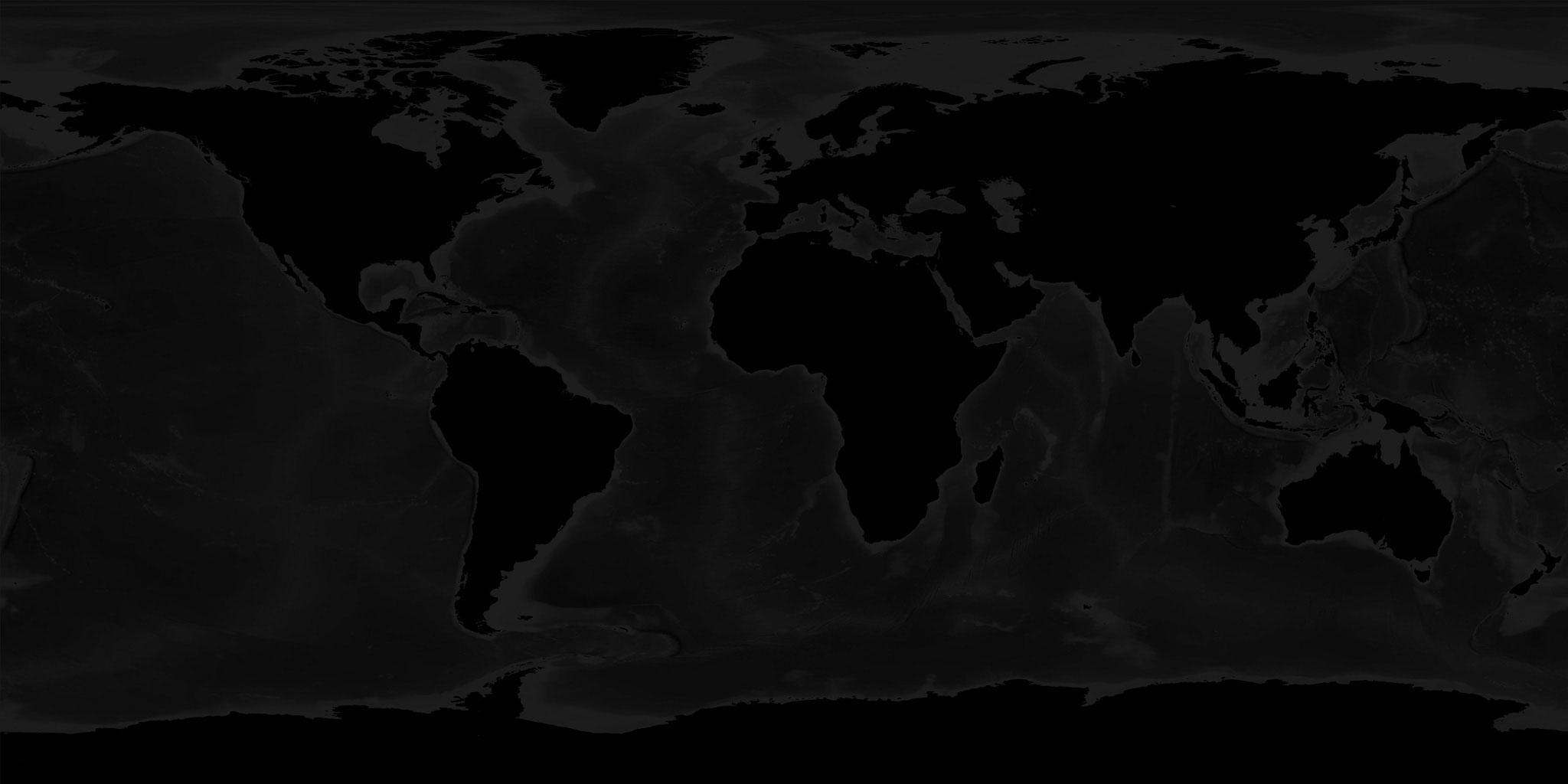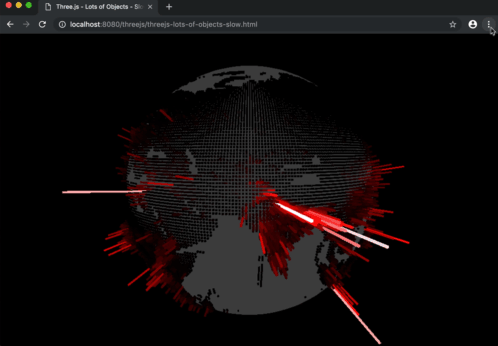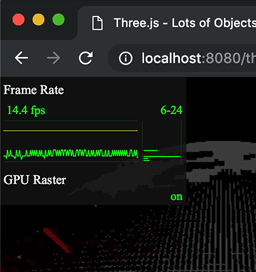# Optimize Lots of Objects

There are many ways to optimize things for three.js. One way is often referred to as merging geometry. Every `Mesh` you create and three.js represents 1 or more requests by the system to draw something. Drawing 2 things has more overhead than drawing 1 even if the results are the same so one way to optimize is to merge meshes.

Let's show an example of when this is a good solution for an issue. Let's re-create the WebGL Globe.

The first thing we need to do is get some data. The WebGL Globe said the data they use comes from SEDAC. Checking out the site I saw there was demographic data in a grid format. I downloaded the data at 60 minute resolution. Then I took a look at the data

It looks like this

``` ncols         360
nrows         145
xllcorner     -180
yllcorner     -60
cellsize      0.99999999999994
NODATA_value  -9999
-9999 -9999 -9999 -9999 -9999 -9999 -9999 -9999 -9999 -9999 -9999 -9999 ...
-9999 -9999 -9999 -9999 -9999 -9999 -9999 -9999 -9999 -9999 -9999 -9999 ...
-9999 -9999 -9999 -9999 -9999 -9999 -9999 -9999 -9999 -9999 -9999 -9999 ...
-9999 -9999 -9999 -9999 -9999 -9999 -9999 -9999 -9999 -9999 -9999 -9999 ...
-9999 -9999 -9999 -9999 -9999 -9999 -9999 -9999 -9999 -9999 -9999 -9999 ...
-9999 -9999 -9999 -9999 -9999 -9999 -9999 -9999 -9999 -9999 -9999 -9999 ...
-9999 -9999 -9999 -9999 -9999 -9999 -9999 -9999 -9999 -9999 -9999 -9999 ...
-9999 -9999 -9999 -9999 -9999 -9999 -9999 -9999 -9999 -9999 -9999 -9999 ...
-9999 -9999 -9999 -9999 -9999 -9999 -9999 -9999 -9999 -9999 -9999 -9999 ...
-9999 -9999 -9999 -9999 -9999 -9999 -9999 -9999 -9999 -9999 -9999 -9999 ...
-9999 -9999 -9999 -9999 -9999 -9999 -9999 -9999 -9999 -9999 -9999 -9999 ...
-9999 -9999 -9999 -9999 -9999 -9999 -9999 -9999 -9999 -9999 -9999 -9999 ...
-9999 -9999 -9999 -9999 -9999 -9999 -9999 -9999 -9999 -9999 -9999 -9999 ...
9.241768 8.790958 2.095345 -9999 0.05114867 -9999 -9999 -9999 -9999 -999...
1.287993 0.4395509 -9999 -9999 -9999 -9999 -9999 -9999 -9999 -9999 -9999...
-9999 -9999 -9999 -9999 -9999 -9999 -9999 -9999 -9999 -9999 -9999 -9999 ...
```

There's a few lines that are like key/value pairs followed by lines with a value per grid point, one line for each row of data points.

To make sure we understand the data let's try to plot it in 2D.

First some code to load the text file

```async function loadFile(url) {
const res = await fetch(url);
return res.text();
}
```

The code above returns a `Promise` with the contents of the file at `url`;

Then we need some code to parse the file

```function parseData(text) {
const data = [];
const settings = {data};
let max;
let min;
// split into lines
text.split('\n').forEach((line) => {
// split the line by whitespace
const parts = line.trim().split(/\s+/);
if (parts.length === 2) {
// only 2 parts, must be a key/value pair
settings[parts] = parseFloat(parts);
} else if (parts.length > 2) {
// more than 2 parts, must be data
const values = parts.map((v) => {
const value = parseFloat(v);
if (value === settings.NODATA_value) {
return undefined;
}
max = Math.max(max === undefined ? value : max, value);
min = Math.min(min === undefined ? value : min, value);
return value;
});
data.push(values);
}
});
return Object.assign(settings, {min, max});
}
```

The code above returns an object with all the key/value pairs from the file as well as a `data` property with all the data in one large array and the `min` and `max` values found in the data.

Then we need some code to draw that data

```function drawData(file) {
const {min, max, data} = file;
const range = max - min;
const ctx = document.querySelector('canvas').getContext('2d');
// make the canvas the same size as the data
ctx.canvas.width = ncols;
ctx.canvas.height = nrows;
// but display it double size so it's not too small
ctx.canvas.style.width = px(ncols * 2);
ctx.canvas.style.height = px(nrows * 2);
// fill the canvas to dark gray
ctx.fillStyle = '#444';
ctx.fillRect(0, 0, ctx.canvas.width, ctx.canvas.height);
// draw each data point
data.forEach((row, latNdx) => {
row.forEach((value, lonNdx) => {
if (value === undefined) {
return;
}
const amount = (value - min) / range;
const hue = 1;
const saturation = 1;
const lightness = amount;
ctx.fillStyle = hsl(hue, saturation, lightness);
ctx.fillRect(lonNdx, latNdx, 1, 1);
});
});
}

function px(v) {
return `\${v | 0}px`;
}

function hsl(h, s, l) {
return `hsl(\${h * 360 | 0},\${s * 100 | 0}%,\${l * 100 | 0}%)`;
}
```

And finally gluing it all together

```loadFile('resources/data/gpw/gpw_v4_basic_demographic_characteristics_rev10_a000_014mt_2010_cntm_1_deg.asc')
.then(parseData)
.then(drawData);
```

Gives us this result

So that seems to work.

Let's try it in 3D. Starting with the code from rendering on demand We'll make one box per data in the file.

First let's make a simple sphere with a texture of the world. Here's the textureAnd the code to set it up.

```{
const geometry = new THREE.SphereGeometry(1, 64, 32);
const material = new THREE.MeshBasicMaterial({map: texture});
}
```

Notice the call to `render` when the texture has finished loading. We need this because we're rendering on demand instead of continuously so we need to render once when the texture is loaded.

Then we need to change the code that drew a dot per data point above to instead make a box per data point.

```function addBoxes(file) {
const {min, max, data} = file;
const range = max - min;

// make one box geometry
const boxWidth = 1;
const boxHeight = 1;
const boxDepth = 1;
const geometry = new THREE.BoxGeometry(boxWidth, boxHeight, boxDepth);
// make it so it scales away from the positive Z axis
geometry.applyMatrix4(new THREE.Matrix4().makeTranslation(0, 0, 0.5));

// these helpers will make it easy to position the boxes
// We can rotate the lon helper on its Y axis to the longitude
const lonHelper = new THREE.Object3D();
// We rotate the latHelper on its X axis to the latitude
const latHelper = new THREE.Object3D();
// The position helper moves the object to the edge of the sphere
const positionHelper = new THREE.Object3D();
positionHelper.position.z = 1;

const lonFudge = Math.PI * .5;
const latFudge = Math.PI * -0.135;
data.forEach((row, latNdx) => {
row.forEach((value, lonNdx) => {
if (value === undefined) {
return;
}
const amount = (value - min) / range;
const material = new THREE.MeshBasicMaterial();
const hue = THREE.MathUtils.lerp(0.7, 0.3, amount);
const saturation = 1;
const lightness = THREE.MathUtils.lerp(0.1, 1.0, amount);
material.color.setHSL(hue, saturation, lightness);
const mesh = new THREE.Mesh(geometry, material);

// adjust the helpers to point to the latitude and longitude
lonHelper.rotation.y = THREE.MathUtils.degToRad(lonNdx + file.xllcorner) + lonFudge;
latHelper.rotation.x = THREE.MathUtils.degToRad(latNdx + file.yllcorner) + latFudge;

// use the world matrix of the position helper to
// position this mesh.
positionHelper.updateWorldMatrix(true, false);
mesh.applyMatrix4(positionHelper.matrixWorld);

mesh.scale.set(0.005, 0.005, THREE.MathUtils.lerp(0.01, 0.5, amount));
});
});
}
```

The code is mostly straight forward from our test drawing code.

We make one box and adjust its center so it scales away from positive Z. If we didn't do this it would scale from the center but we want them to grow away from the origin.

default

Of course we could also solve that by parenting the box to more `THREE.Object3D` objects like we covered in scene graphs but the more nodes we add to a scene graph the slower it gets.

We also setup this small hierarchy of nodes of `lonHelper`, `latHelper`, and `positionHelper`. We use these objects to compute a position around the sphere were to place the box.

Above the green bar represents `lonHelper` and is used to rotate toward longitude on the equator. The blue bar represents `latHelper` which is used to rotate to a latitude above or below the equator. The red sphere represents the offset that that `positionHelper` provides.

We could do all of the math manually to figure out positions on the globe but doing it this way leaves most of the math to the library itself so we don't need to deal with.

For each data point we create a `MeshBasicMaterial` and a `Mesh` and then we ask for the world matrix of the `positionHelper` and apply that to the new `Mesh`. Finally we scale the mesh at its new position.

Like above, we could also have created a `latHelper`, `lonHelper`, and `positionHelper` for every new box but that would be even slower.

There are up to 360x145 boxes we're going to create. That's up to 52000 boxes. Because some data points are marked as "NO_DATA" the actual number of boxes we're going to create is around 19000. If we added 3 extra helper objects per box that would be nearly 80000 scene graph nodes that THREE.js would have to compute positions for. By instead using one set of helpers to just position the meshes we save around 60000 operations.

A note about `lonFudge` and `latFudge`. `lonFudge` is π/2 which is a quarter of a turn. That makes sense. It just means the texture or texture coordinates start at a different offset around the globe. `latFudge` on the other hand I have no idea why it needs to be π * -0.135, that's just an amount that made the boxes line up with the texture.

The last thing we need to do is call our loader

```loadFile('resources/data/gpw/gpw_v4_basic_demographic_characteristics_rev10_a000_014mt_2010_cntm_1_deg.asc')
.then(parseData)
-  .then(drawData)
+  .then(render);
```

Once the data has finished loading and parsing then we need to render at least once since we're rendering on demand.

If you try to rotate the example above by dragging on the sample you'll likely notice it's slow.

We can check the framerate by opening the devtools and turning on the browser's frame rate meter.On my machine I see a framerate under 20fps.That doesn't feel very good to me and I suspect many people have slower machines which would make it even worse. We'd better look into optimizing.

For this particular problem we can merge all the boxes into a single geometry. We're currently drawing around 19000 boxes. By merging them into a single geometry we'd remove 18999 operations.

Here's the new code to merge the boxes into a single geometry.

```function addBoxes(file) {
const {min, max, data} = file;
const range = max - min;

-  // make one box geometry
-  const boxWidth = 1;
-  const boxHeight = 1;
-  const boxDepth = 1;
-  const geometry = new THREE.BoxGeometry(boxWidth, boxHeight, boxDepth);
-  // make it so it scales away from the positive Z axis
-  geometry.applyMatrix4(new THREE.Matrix4().makeTranslation(0, 0, 0.5));

// these helpers will make it easy to position the boxes
// We can rotate the lon helper on its Y axis to the longitude
const lonHelper = new THREE.Object3D();
// We rotate the latHelper on its X axis to the latitude
const latHelper = new THREE.Object3D();
// The position helper moves the object to the edge of the sphere
const positionHelper = new THREE.Object3D();
positionHelper.position.z = 1;
+  // Used to move the center of the box so it scales from the position Z axis
+  const originHelper = new THREE.Object3D();
+  originHelper.position.z = 0.5;

const lonFudge = Math.PI * .5;
const latFudge = Math.PI * -0.135;
+  const geometries = [];
data.forEach((row, latNdx) => {
row.forEach((value, lonNdx) => {
if (value === undefined) {
return;
}
const amount = (value - min) / range;

-      const material = new THREE.MeshBasicMaterial();
-      const hue = THREE.MathUtils.lerp(0.7, 0.3, amount);
-      const saturation = 1;
-      const lightness = THREE.MathUtils.lerp(0.1, 1.0, amount);
-      material.color.setHSL(hue, saturation, lightness);
-      const mesh = new THREE.Mesh(geometry, material);

+      const boxWidth = 1;
+      const boxHeight = 1;
+      const boxDepth = 1;
+      const geometry = new THREE.BoxGeometry(boxWidth, boxHeight, boxDepth);

// adjust the helpers to point to the latitude and longitude
lonHelper.rotation.y = THREE.MathUtils.degToRad(lonNdx + file.xllcorner) + lonFudge;
latHelper.rotation.x = THREE.MathUtils.degToRad(latNdx + file.yllcorner) + latFudge;

-      // use the world matrix of the position helper to
-      // position this mesh.
-      positionHelper.updateWorldMatrix(true, false);
-      mesh.applyMatrix4(positionHelper.matrixWorld);
-
-      mesh.scale.set(0.005, 0.005, THREE.MathUtils.lerp(0.01, 0.5, amount));

+      // use the world matrix of the origin helper to
+      // position this geometry
+      positionHelper.scale.set(0.005, 0.005, THREE.MathUtils.lerp(0.01, 0.5, amount));
+      originHelper.updateWorldMatrix(true, false);
+      geometry.applyMatrix4(originHelper.matrixWorld);
+
+      geometries.push(geometry);
});
});

+  const mergedGeometry = BufferGeometryUtils.mergeBufferGeometries(
+      geometries, false);
+  const material = new THREE.MeshBasicMaterial({color:'red'});
+  const mesh = new THREE.Mesh(mergedGeometry, material);

}
```

Above we removed the code that was changing the box geometry's center point and are instead doing it by adding an `originHelper`. Before we were using the same geometry 19000 times. This time we are creating new geometry for every single box and since we are going to use `applyMatrix` to move the vertices of each box geometry we might as well do it once instead of twice.

At the end we pass an array of all the geometries to `BufferGeometryUtils.mergeBufferGeometries` which will combined all of them into a single mesh.

We also need to include the `BufferGeometryUtils`

```import * as BufferGeometryUtils from '/examples/jsm/utils/BufferGeometryUtils.js';
```

And now, at least on my machine, I get 60 frames per second

So that worked but because it's one mesh we only get one material which means we only get one color where as before we had a different color on each box. We can fix that by using vertex colors.

Vertex colors add a color per vertex. By setting all the colors of each vertex of each box to specific colors every box will have a different color.

```+const color = new THREE.Color();

const lonFudge = Math.PI * .5;
const latFudge = Math.PI * -0.135;
const geometries = [];
data.forEach((row, latNdx) => {
row.forEach((value, lonNdx) => {
if (value === undefined) {
return;
}
const amount = (value - min) / range;

const boxWidth = 1;
const boxHeight = 1;
const boxDepth = 1;
const geometry = new THREE.BoxGeometry(boxWidth, boxHeight, boxDepth);

// adjust the helpers to point to the latitude and longitude
lonHelper.rotation.y = THREE.MathUtils.degToRad(lonNdx + file.xllcorner) + lonFudge;
latHelper.rotation.x = THREE.MathUtils.degToRad(latNdx + file.yllcorner) + latFudge;

// use the world matrix of the origin helper to
// position this geometry
positionHelper.scale.set(0.005, 0.005, THREE.MathUtils.lerp(0.01, 0.5, amount));
originHelper.updateWorldMatrix(true, false);
geometry.applyMatrix4(originHelper.matrixWorld);

+    // compute a color
+    const hue = THREE.MathUtils.lerp(0.7, 0.3, amount);
+    const saturation = 1;
+    const lightness = THREE.MathUtils.lerp(0.4, 1.0, amount);
+    color.setHSL(hue, saturation, lightness);
+    // get the colors as an array of values from 0 to 255
+    const rgb = color.toArray().map(v => v * 255);
+
+    // make an array to store colors for each vertex
+    const numVerts = geometry.getAttribute('position').count;
+    const itemSize = 3;  // r, g, b
+    const colors = new Uint8Array(itemSize * numVerts);
+
+    // copy the color into the colors array for each vertex
+    colors.forEach((v, ndx) => {
+      colors[ndx] = rgb[ndx % 3];
+    });
+
+    const normalized = true;
+    const colorAttrib = new THREE.BufferAttribute(colors, itemSize, normalized);
+    geometry.setAttribute('color', colorAttrib);

geometries.push(geometry);
});
});
```

The code above looks up the number or vertices needed by getting the `position` attribute from the geometry. We then create a `Uint8Array` to put the colors in. It then adds that as an attribute by calling `geometry.setAttribute`.

Lastly we need to tell three.js to use the vertex colors.

```const mergedGeometry = BufferGeometryUtils.mergeBufferGeometries(
geometries, false);
-const material = new THREE.MeshBasicMaterial({color:'red'});
+const material = new THREE.MeshBasicMaterial({
+  vertexColors: true,
+});
const mesh = new THREE.Mesh(mergedGeometry, material);